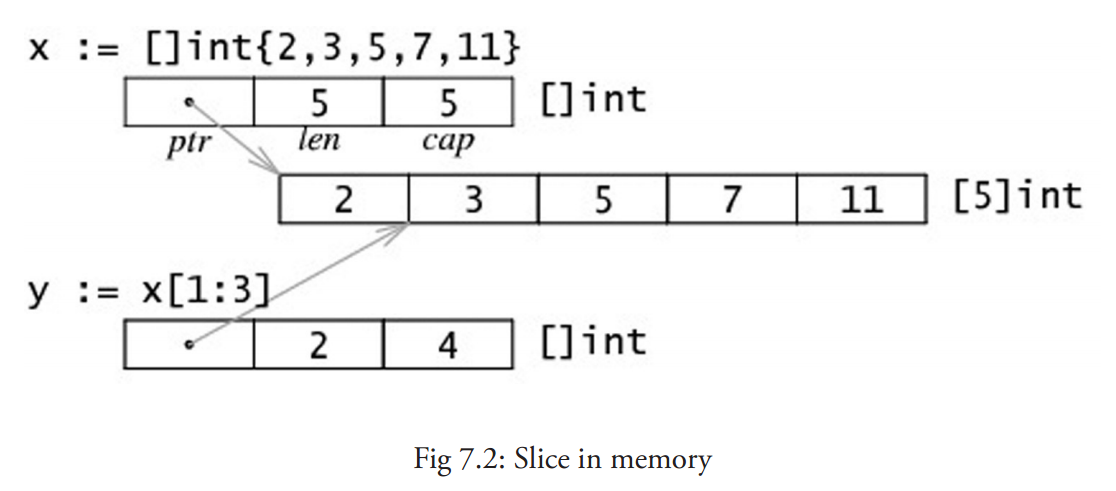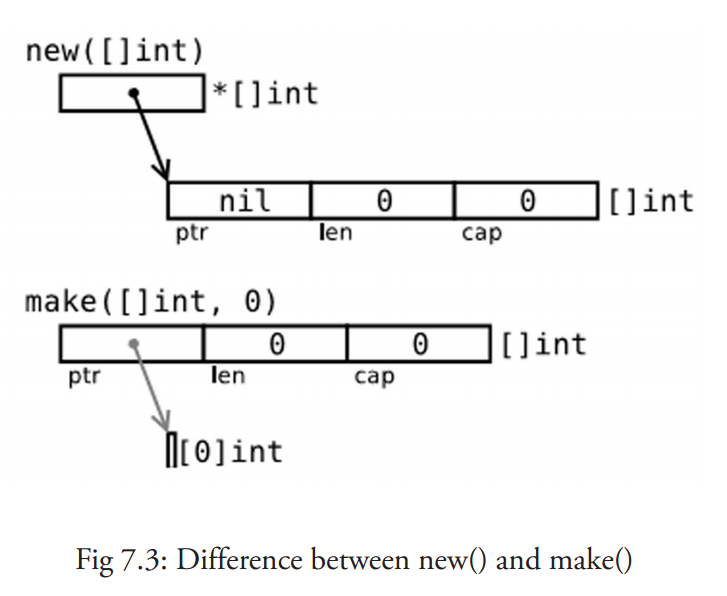# 7.2 切片

## 7.2.1 概念

`arr1[2:]``arr1[2:len(arr1)]` 相同，都包含了数组从第三个到最后的所有元素。

`arr1[:3]``arr1[0:3]` 相同，包含了从第一个到第三个元素（不包括第三个）。

`s2 := s[:]` 是用切片组成的切片，拥有相同的元素，但是仍然指向相同的相关数组。

``````s == s[:i] + s[i:] // i是一个整数且: 0 <= i <= len(s)
len(s) < cap(s)
``````

• `y = 3``y = 5`
• 切片 `y[0:4]` 由 元素 3, 5， 7 和 11 组成。``````package main
import "fmt"

func main() {
var arr1 int
var slice1 []int = arr1[2:5] // item at index 5 not included!

// load the array with integers: 0,1,2,3,4,5
for i := 0; i < len(arr1); i++ {
arr1[i] = i
}

// print the slice
for i := 0; i < len(slice1); i++ {
fmt.Printf("Slice at %d is %d\n", i, slice1[i])
}

fmt.Printf("The length of arr1 is %d\n", len(arr1))
fmt.Printf("The length of slice1 is %d\n", len(slice1))
fmt.Printf("The capacity of slice1 is %d\n", cap(slice1))

// grow the slice
slice1 = slice1[0:4]
for i := 0; i < len(slice1); i++ {
fmt.Printf("Slice at %d is %d\n", i, slice1[i])
}
fmt.Printf("The length of slice1 is %d\n", len(slice1))
fmt.Printf("The capacity of slice1 is %d\n", cap(slice1))

// grow the slice beyond capacity
//slice1 = slice1[0:7 ] // panic: runtime error: slice bound out of range
}
``````

``````Slice at 0 is 2
Slice at 1 is 3
Slice at 2 is 4
The length of arr1 is 6
The length of slice1 is 3
The capacity of slice1 is 4
Slice at 0 is 2
Slice at 1 is 3
Slice at 2 is 4
Slice at 3 is 5
The length of slice1 is 4
The capacity of slice1 is 4
``````

## 7.2.2 将切片传递给函数

``````func sum(a []int) int {
s := 0
for i := 0; i < len(a); i++ {
s += a[i]
}
return s
}

func main() {
var arr = int{0, 1, 2, 3, 4}
sum(arr[:])
}
``````

## 7.2.3 用 make() 创建一个切片

make 接受 2 个参数：元素的类型以及切片的元素个数。

make 的使用方式是：`func make([]T, len, cap)`，其中 cap 是可选参数。

``````make([]int, 50, 100)
new(int)[0:50]
``````

``````package main
import "fmt"

func main() {
var slice1 []int = make([]int, 10)
for i := 0; i < len(slice1); i++ {
slice1[i] = 5 * i
}

// print the slice:
for i := 0; i < len(slice1); i++ {
fmt.Printf("Slice at %d is %d\n", i, slice1[i])
}
fmt.Printf("\nThe length of slice1 is %d\n", len(slice1))
fmt.Printf("The capacity of slice1 is %d\n", cap(slice1))
}
``````

``````Slice at 0 is 0
Slice at 1 is 5
Slice at 2 is 10
Slice at 3 is 15
Slice at 4 is 20
Slice at 5 is 25
Slice at 6 is 30
Slice at 7 is 35
Slice at 8 is 40
Slice at 9 is 45
The length of slice1 is 10
The capacity of slice1 is 10
``````

## 7.2.4 new() 和 make() 的区别

• new(T) 为每个新的类型T分配一片内存，初始化为 0 并且返回类型为*T的内存地址：这种方法 返回一个指向类型为 T，值为 0 的地址的指针，它适用于值类型如数组和结构体（参见第 10 章）；它相当于 `&T{}`
• make(T) 返回一个类型为 T 的初始值，它只适用于3种内建的引用类型：切片、map 和 channel（参见第 8 章，第 13 章）。``````var p *[]int = new([]int) // *p == nil; with len and cap 0
p := new([]int)
``````

``````var v []int = make([]int, 10, 50)
``````

``````v := make([]int, 10, 50)
``````

## 7.2.6 bytes 包

bytes 包和字符串包十分类似（参见第 4.7 节）。而且它还包含一个十分有用的类型 Buffer:

``````import "bytes"

type Buffer struct {
...
}
``````

Buffer 可以这样定义：`var buffer bytes.Buffer`

``````var buffer bytes.Buffer
for {
if s, ok := getNextString(); ok { //method getNextString() not shown here
buffer.WriteString(s)
} else {
break
}
}
fmt.Print(buffer.String(), "\n")
``````